Next: Resonance Mirror Up: Experimental Approach and Equipment Previous: Loss Monitors   Contents

## Finding the Depolarizing Resonance

The high precision of this resonant depolarization experiment is based on the fact that the energy measurement is actually a frequency measurement. Frequency measurements are simple, quick and very precise. Recall equation 14 describing the spin tune: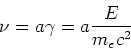(49)

The design energy of the SLS storage ring is 2.4 GeV which leads to an estimated spin tune of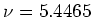. The resonant depolarizing frequency is the fractional part of the spin tune multiplied with the revolution frequency: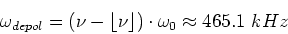(50)

So we expect to find the resonant depolarizing frequency around 465 kHz. Form this one is able to calculate the energy: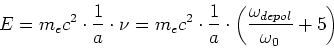(51)

The resonance is found by means of sweeping over a frequency range around the expected value determining the precise frequency at which the beam gets depolarized.

This is done by using a signal generator which feeds a sine wave to the input of the kicker magnet amplifier. The frequency of this sine wave is swept over a pre-defined interval with a certain sweep velocity or dwell (the time span in which a sine wave of a certain frequency is fed to the amplifier). For example we could start at 400 kHz and sweep until we reach 500 kHz with a step size of 1 Hz and a dwell of 1 s meaning that upon triggering the sweep the signal generator will feed a sine wave with 400 kHz frequency to the amplifier and will raise this value linearly by one Hertz per second until it reaches 500 kHz7. Observing when the resonant depolarization of the beam occurs the actual frequency is recorded and the beam energy calculated according to equation 51.

Since we are using very low frequencies (the signal generator supports frequencies up to 1.1 Ghz) and we only make use of large dwells (the signal generator is capable of ms dwells) the generator is very precise; measurements of the generator output on an oscilloscope showed that the generator fed exactly the required frequency to the amplifier with a precision much higher than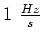. It is important to note here that the uncertainty of the frequency generator's output frequency has a negligible influence on the uncertainty of this measurement because the frequency uncertainty is magnitudes smaller than other effects; these will be discussed later.Next: Resonance Mirror Up: Experimental Approach and Equipment Previous: Loss Monitors   Contents
Simon Leemann
2002-03-15